RS Aggarwal Class 7 Solutions Chapter 7 - Linear Equations In One Variable

RS Aggarwal Class 7 Chapter 7 - Linear Equations In One Variable Solutions Free PDF

An equation in mathematics which contains only one variable along with the respective real numbers and coefficients is called as a Linear equation in one variable. The variable is always unknown in this case and by producing a solution for this problem, we can effectively solve for it. Engineering and Physics are the fields where the maximum utilization of Linear equations occurs.

A linear equation in one variable can be represented as an equation in the form: ax+b=0

Some of the various forms linear equations may take are:

1. Matrix Form
2. Parametric Form
3. Two Variables
4. Two point form
5. Cartesian Coordinates

The RS Aggarwal Class 7 Solutions Chapter 7 Linear Equations in One Variable can be found below: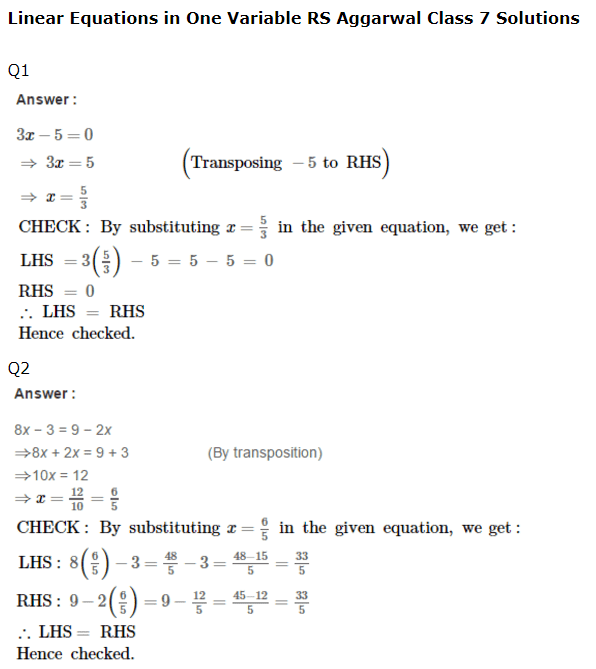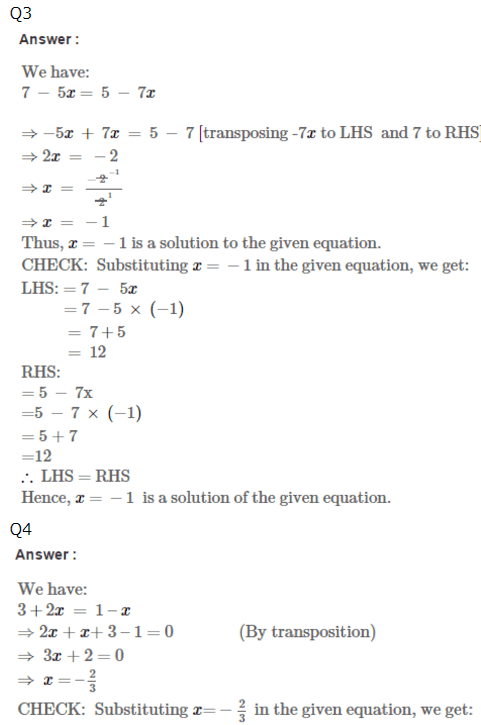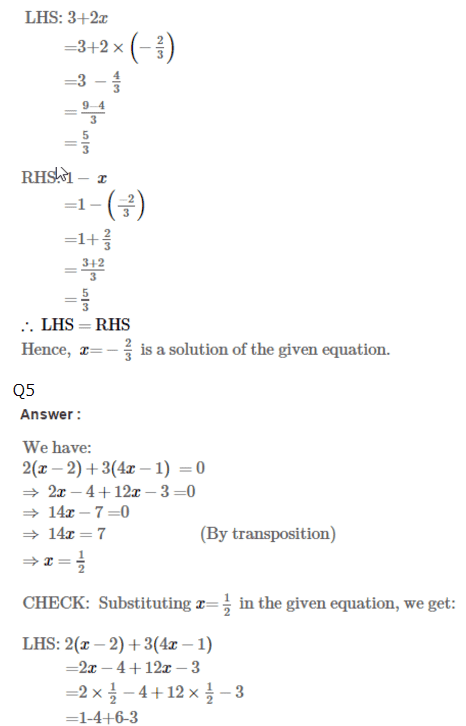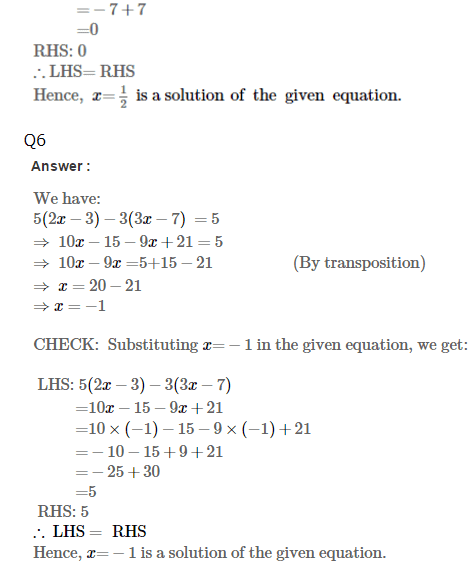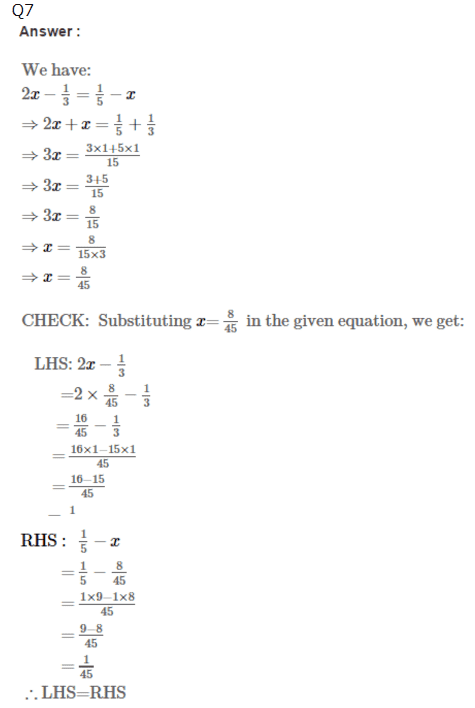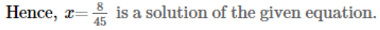Practise This Question

If I sell 45th of 10 apples, how many are left?